# 1729

## Summary

### Names

This number is called the Hardy-Ramanujan number after a conversation between Hardy and Ramanujan where Ramanujan observed that it is the smallest number expressible as the sum of two cubes in two distinct ways: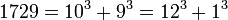.

### Factorization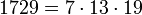### Properties and families

Property or family Parameter values First few members Proof of membership/containment/satisfaction
Carmichael number third among them 561, 1105, 1729, 2465, 2821, 6601, [SHOW MORE]View list on OEIS The universal exponent is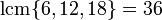which divides 1728.
Poulet number (Fermat pseudoprime to base 2) sixth among them 341, 561, 645, 1105, 1387, 1729, 1905, 2047, [SHOW MORE] View list on OEIS follows from being a Carmichael number.

## Arithmetic functions

Function Value Explanation
Euler totient function 1296 It is the product.
universal exponent 36 It is the least common multiple of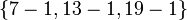.
divisor count function 8 It is the product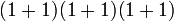where the first 1s in each sum represent the multiplicities of the prime divisors.
divisor sum function 2240 It is the product of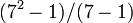,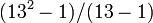, and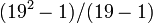largest prime divisor 19 direct from factorization
largest prime power divisor 19 direct from factorization
square-free part 1729 the original number is a square-free number.
Mobius function -1 the number is square-free and has an odd number of prime divisors (namely, 3 prime divisors).